## How to Calculate and Solve for the Volume, Radius and Height of a Cylinder | Nickzom Calculator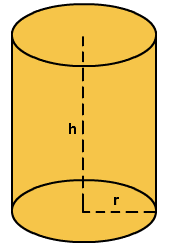The image above represents a cylinder.
To compute the volume of a cylinder requires two essential parameters which are the radius and height of the cylinder.

The formula for computing the volume of a cylinder is:

V = πr2h

Where:
V = Volume of a cylinder
r = radius of the cylinder
h = height of the cylinder

Let’s solve an example
Find the volume of a cylinder with a radius of 3 cm and a height of 5 cm.

This implies that:
r = radius of the cylinder = 3
h = height of the cylinder = 5

V = πr2h
V = 3.142 x 32 x 5
V = 141.39

Therefore, the volume of the cylinder is 141.39 cm3.

Calculating the Height of a cylinder when Volume and Radius is Given

The formula is h = V / πr2

Where;
V = Volume of a cylinder
r =  radius of the cylinder
h = height of the cylinder

Let’s solve an example:
Find the height of a cylinder with a volume of 300 cm3 and a radius of 3 cm

This implies that;
V = Volume of the cylinder = 300 cm3
r  = radius of the cylinder = 3 cm

h = V / πr2
h = 300 / 3.142(3)2
h = 300 / 28.278
h = 10.61
Therefore, the height of the cylinder is 10.61 cm.

Calculating the Radius of a cylinder when Volume and Height is Given

The formula is r = √(V / πh)

Where;
V = Volume of a cylinder
r =  radius of the cylinder
h = height of the cylinder

Let’s solve an example:
Find the radius of a cylinder with a volume of 200 cm3 and a height of 5 cm

This implies that;
V = Volume of the cylinder = 200 cm3
h  = height of the cylinder = 5 cm

r = √(V / πh)
r = √(200 / 3.142(5))
r = √(200 / 15.71)
r = √12.73
r = 3.57

Therefore, the radius of the cylinder is 3.57 cm.

## How to Calculate the Area, Radius and Height of a Cylinder | Nickzom Calculator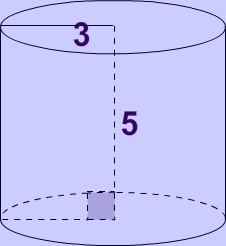The image above represents a cylinder.
To compute the area of a cylinder requires two essential parameters which are the radius and height of the cylinder.

The formula for computing the area of a cylinder is:

A = 2πrh + 2πr2

Where:
A = Area of a cylinder
r = radius of the cylinder
h = height of the cylinder

Let’s solve an example
Find the area of a cylinder with a radius of 3 cm and a height of 5 cm.

This implies that:
r = radius of the cylinder = 3
h = height of the cylinder = 5

A = 2πrh + 2πr2
A = 2(3.142) x 3 x 5 + 2(3.142) x 32
A = 150.82

Therefore, the area of the cylinder is 150.82 cm2.

Calculating the Height of a cylinder when Area and Radius is Given

The formula is h =  A/ 2πr – r

Where;
A = Area of a cylinder
r =  radius of the cylinder
h = height of the cylinder

Let’s solve an example:
Find the height of a cylinder with an area of 600 cm² and a radius of 5 cm

This implies that;
A = Area of the cylinder = 600 cm²
r  = radius of the cylinder = 5 cm

h = A / 2πr – r
h = 600 / 2(3.142)(5) – 5
h = 600 / 31.42 – 5
h = 19.10 – 5
h = 14.10

Therefore, the height of the cylinder is 14.10 cm.

## How to Calculate and Solve for the Perimeter or Circumference, Radius and Diameter of a Circle | The Calculator Encyclopedia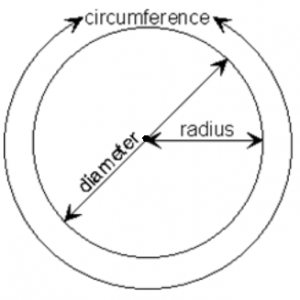The image above is a circle.

To compute the perimeter or circumference of a circle, one essential parameter is needed and this parameter is the radius of a circle (r). You can also use the diameter of a circle to compute the area of a circle (d).

The formula for calculating the perimeter or circumference of a circle is:

P = 2πr

Where:

P = Perimeter or Circumference of a circle
r = Radius of a circle

Let’s solve an example:
Find the perimeter or circumference of a circle where the radius of a circle is 8 cm.

This implies that;
r = Radius of a circle = 8 cm

P = 2πr
P = 2 x 3.142 x 8
P = 50.265

Therefore, the perimeter or circumference of a circle is 50.265 cm.

Calculating the Area of a Circle using the Diameter of a Circle.

The formula is P = πd

Where:

P = Perimeter or Circumference of a circle
d = Diameter of a circle

Let’s solve an example:
Find the perimeter or circumference of a circle where the diameter of a circle is 10 cm.

This implies that;
d = Diameter of a circle = 10 cm

P = πd
P = 3.142 x 10
P = 31.42

Therefore, the perimeter or circumference of a circle with diameter is 31.42 cm.

How to Calculate Radius of a Circle when Perimeter or Circumference of the Circle is Given

r = P /

where;

r = Radius of a circle
P = Perimeter or Circumference of a circle

Let’s solve an example:
Find the radius of a circle where the perimeter or circumference of the circle is 16 cm.

This implies that;
P = Perimeter or Circumference of the circle = 16 cm

r = P /
r = 16 / 6.284
r = 2.55

Therefore, the radius of the circle is 2.55 cm.

How to Calculate Diameter of a Circle when Perimeter or Circumference of the Circle is Given

d = P / π

where;

d = Diameter of a circle
P = Perimeter or Circumference of a circle

Let’s solve an example;
Find the diameter of a circle where the perimeter or circumference of the circle is 20 cm

This implies that;
P = Perimeter or Circumference of the circle = 20 cm

d = P / π
d = 20 / π
d = 6.365

Therefore, the diameter of the circle is 6.365 cm.

## How To Calculate and Solve for the Area, Radius and Diameter of a Circle | The Calculator Encyclopedia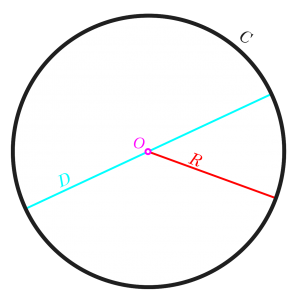The image above is a circle.

To compute the area of a circle, one essential parameter is needed and this parameter is the radius of a circle (r). You can also use the diameter of a circle to compute the area of a circle (d).

The formula for calculating the area of a circle is:

A = πr2

Where:

A = Area of a circle
r = Radius of a circle

Let’s solve an example:
Find the area of a circle where the radius of a circle is 6 cm.

This implies that;
r = Radius of a circle = 6 cm

A = πr2
A = 3.142 (6)2
A = 3.142 (36)
A = 113.10

Therefore, the area of a circle is 113.10 cm2

Calculating the Area of a Circle using the Diameter of a Circle.

The formula is A = πd2/4

Where;
A = Area of a circle
d = Diameter of a circle

Let’s solve an example:
Find the area of a circle where the diameter of the circle is 7 cm.

This implies that;
d = Diameter of a circle = 4 cm

A = πd2 / 4
A = 3.142 (4)2 / 4
A = 3.142 (16) / 4
A = 3.142 (4)
A = 12.57

Therefore, the area of a circle with diameter given is 12.57 cm2

## How to Calculate and Solve the Centre of Gravity of a Segment of a Sphere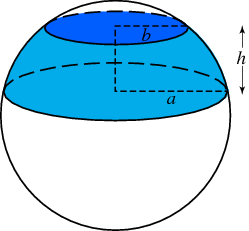The image above represents a segment of a sphere.
To compute the centre of gravity of a segment of a sphere requires two essential parameters. These parameters are the radius of the sphere and height of the segment of the sphere.

The formula for computing the centre of gravity of a sphere is:

C.G. = 3(2r – h)² / 4(3r – h)

Where:
C.G. = Centre of Gravity
r = Radius of the Sphere
h = Height of the Segment of the Sphere

Let’s solve an example
Find the centre of gravity of the segment of the sphere where the radius of the sphere is 10 m and the height of the segment of the sphere is 4 m.

This implies that:
r = Radius of the Sphere = 10
h = Height of the Segment of the Sphere = 4

C.G. = 3(2(10) – 4)² / 4(3(10) – 4)
C.G. = 3(20 – 4)² / 4(30 – 4)
C.G. = 3(16)² / 4(26)
C.G. = 3(256) / 104
C.G. = 768 / 104
C.G. = 7.38

Therefore, the centre of gravity of the segment of the sphere is 7.38.

Nickzom Calculator – The Calculator Encyclopedia is capable of calculating the centre of gravity of a segment of a sphere at a height, h at a distance from the centre of the sphere measured along the height.

## How to Calculate and Solve for the Centroid or Centre of Gravity of a HemisphereThe image above is a hemisphere with a radius of 5.
To compute the centroid or centre of gravity of a hemisphere. You need one essential parameter and this parameter is the radius of the hemisphere (r).

The formula for calculating the centroid or centre of gravity of a hemisphere is:

C.G. = 3r / 8

Where
r = Radius of the hemisphere

As always let us try and solve an example:
Find the centroid or centre of gravity of a hemisphere where the radius is 5 cm.

From the formula this implies that:
r = Radius of the hemisphere = 5

C.G. = 3(5) / 8
C.G. = 15 / 8
C.G. = 1.875

Therefore, the centroid or centre of gravity of the hemisphere is 1.875.

Nickzom Calculator – The Calculator Encyclopedia is capable of calculating the centre of gravity of a hemisphere at a distance from its base measured along the vertical radius.

To get the answer and workings of the center of gravity or centroid of a hemisphere using the Nickzom Calculator – The Calculator Encyclopedia. First, you need to obtain the app.

You can get this app via any of these means:

## Nickzom Calculator Calculates the Centroid or Centre of Gravity of a Semicircle | Statics (Mechanics)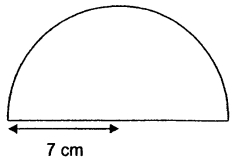The image above is a semicircle with a radius of 7 cm.
To compute the centroid or centre of gravity of a semicircle, you need one essential parameter and this parameter is the radius of the semicircle.

The formula for calculating the centroid or centre of gravity of a semicircle is:

C.G.= 4r /

Where:
r = Radius of the Semicircle
π = Mathematical Constant = 3.142 (approximately)

Let’s solve an example:
Find the centroid or centre of gravity of a semicircle where the radius is 7 cm.

From the example above,
r = radius of the semicircle = 7

C.G. = 4(7) /
C.G. = 28 / 9.4247
C.G. = 2.97

Therefore, the centroid or centre of gravity of the semicircle is 2.97.

Nickzom Calculator – The Calculator Encyclopedia is capable of calculating the centre of gravity of a semicircle at a distance from its base measured along the vertical radius.

To get the answer and workings of the center of gravity or centroid of a semicircle using the Nickzom Calculator – The Calculator Encyclopedia. First, you need to obtain the app.